• 研究了具有零相关区的高斯整数序列集构造方法。该方法基于二元正交矩阵，首先利用插零法构造出具有零相关区的三元序列集。然后利用完备高斯整数序列进行滤波，从而将三元序列变换成高斯整数序列且保持序列相关函数值...正交矩阵
•研究论文
• 主要讨论了高斯整数多项式所表整数的计算，利用初等数论基本理论和方法，获得了一个含有n（n≥1）个高斯整数常量和变量的线性多项式虚部所表整数中最小正整数的精确显式表达，并进一步获得了该多项式虚部所表整数的...
• 高斯整数 a=x+y∗i  (x,y∈Z)a = x+y*i\ \ (x,y\in Z)a=x+y∗i  (x,y∈Z)，则 aaa 为高斯整数。 aaa 的范为 N(a)=∣a2∣=x2+y2N(a)=|a^2|=x^2+y^2N(a)=∣a2∣=x2+y2。 若存在高斯整数 yyy，...
前言
如果你对这篇文章可感兴趣，可以点击「【访客必读 - 指引页】一文囊括主页内所有高质量博客」，查看完整博客分类与对应链接。
高斯整数

a

=

x

+

y

∗

i

(

x

,

y

∈

Z

)

a = x+y*i\ \ (x,y\in Z)

，则

a

a

为高斯整数。

a

a

的范为

N

(

a

)

=

∣

a

2

∣

=

x

2

+

y

2

N(a)=|a^2|=x^2+y^2

。若存在高斯整数

y

y

，使得

a

y

=

1

ay=1

，则

a

a

为高斯整数中的乘法可逆元，

y

y

为

a

a

的逆。高斯整数

a

a

是可逆元的充要条件是

N

(

a

)

=

1

N(a)=1

，高斯整数中只有

4

4

个可逆元，分别是

−

1

、

1

、

i

、

−

i

-1、1、i、-i

。

a

、

b

a、b

为高斯整数，

a

=

b

y

a=by

，则

a

a

和

b

b

等价，即

a

=

b

、

−

b

、

i

b

、

−

i

b

a=b、-b、ib、-ib

。

高斯素数
定义：设

ϕ

\phi

为高斯整数中的非零非可逆元，则

ϕ

\phi

为高斯素数。即

ϕ

\phi

的因子或者为可逆元，或者是与

ϕ

\phi

等价的高斯整数。

ϕ

\phi

为高斯整数，且

N

(

ϕ

)

=

p

N(\phi)=p

为素数，则

ϕ

\phi

必定为高斯素数。若

ϕ

\phi

为高斯素数，则其共轭元也是高斯素数。

高斯素数判断
高斯整数

a

+

b

i

a+bi

是素数，当且仅当：

a

、

b

a、b

中有一个是零，另一个数的绝对值是形如

4

∗

n

+

3

4*n+3

的素数。

a

、

b

a、b

均不为零，而

a

2

+

b

2

a^2+b^2

为素数。

费马平方和定理
奇质数能表示为两个平方数之和的充分必要条件是该质数被

4

4

除余

1

1

，即

4

∗

n

+

1

4*n+1

。例题：找出

[

l

,

r

]

[l,r]

中的素数

t

t

，满足

t

=

a

2

+

b

2

(

a

,

b

∈

N

∗

)

t=a^2+b^2\ \ (a,b\in N^{*})

，输出这种素数总数。
展开全文高斯素数 费马平方和定理
• 提出一类基于分圆类构造完备高斯整数序列的方法。分别通过有限域GF(p)上的2阶和4阶分圆类，构造得到自由度分别为3和5的高斯整数序列，序列长度为奇素数，该序列具有良好的完备自相关性能。该构造方法解决了以往利用...伪随机序列 离散傅里叶变换
•研究论文
• 一、高斯整数 形如。（其中a，b是整数）的复数被称为高斯整数高斯整数全体记作Z[i]。注意到若 γ=a+bi 是高斯整数，则它是满足如下方程的代数整数： 通常我们使用希腊字母来表示高斯整数，例如α，β，γ和δ...
一、高斯整数
形如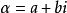。（其中a，b是整数）的复数被称为高斯整数，高斯整数全体记作Z[i]。注意到若 γ=a+bi 是高斯整数，则它是满足如下方程的代数整数：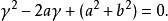通常我们使用希腊字母来表示高斯整数，例如α，β，γ和δ。注意到若 n 是一个整数，则 n=n+0i 也是高斯整数。当我们讨论高斯整数的时候，把通常的整数称为有理整数。
加、减、乘运算
高斯整数在加、减、乘运算下是封闭的，正如下面定理所述。
定理1：设 α=x+iy 和 β=w+iz 是高斯整数，其中 x，y，w 和 z 是有理整数，则 α+β，α-β 和 αβ 都是高斯整数。
虽然高斯整数在加、减和乘运算下封闭，但是他们在除法运算下并不封闭，这一点与有理整数类似。此外，若 α=a+bi 是高斯整数，则a的范数 N(α)= 是非负有理整数。

整除性
我们可以像研究有理整数那样去研究高斯整数。整数的许多基本性质可以直接类推到高斯整数上。要讨论高斯整数的这些性质，我们需要介绍高斯整数类似于通常整数的一些概念。特别地，我们需要说明一个高斯整数整除另一个高斯整数的意义，并给出高斯素数的定义。
定义1：设 α 和 β 是高斯整数，我们称α整除β，是指存在一个高斯整数 γ 使得β=αγ。若α整除β，我们记作α|β ；若α 不整除β ，记作αβ 。
高斯整数的整除也满足有理整数整除的一些相同的性质。例如，若α，β和γ 是高斯整数，α|β，β|γ，则α|γ。再者，若α，β，γ，ν和μ 是高斯整数，γ|μ，γ|β，则γ|(μα+νβ)。
高斯素数
1, −1, i及−i都是高斯整数环里面的单位元。除此之外，在高斯整环里面不能因子分解的数称为高斯素数。高斯素数分为两类，其中一类是形式为4n+3（n是整数）的普通素数，如3，7等，它们在高斯整环里面也不能够因子分解。但是所有形式是4n+1的普通素数如5，13等，在高斯整环里面都可以唯一因子分解成两个共轭的高斯素数的乘积，如5=(2+i)(2-i)。需要注意的是，这里我们也可以写成5=(1+2i)(1-2i)，这个是因为(2-i)i=1+2i，而i是单位元，所以我们可以认为这两种分解是等价的。此外，素数2也可以分解，即2=(1+i)(1-i)。

二、费马平方和定理
费马平方和定理是指由法国数学家费马在1640年提出的一个猜想，但他没有提出有力的数学证明，1747年，瑞士数学家莱昂哈德•欧拉提出证明后成为定理。
费马平方和定理的表述是：奇质数能表示为两个平方数之和的充分必要条件是该素数被4除余1。
费马二平方定理(Fermat's Two Squares Theorem)
费马二平方定理是指除了2这个特殊的素数，所有的素数都可以分两类：被4除余1的素数，如5，13，17，29，37，41；第二类则是被4除余3的素数如3，7，11，19，23，31.第一类素数都能表示为两个整数的平方和，第二类都不能。
费马二平方定理指出，当且仅当或时，素数可以表示为两个非零平方之和。 并且这种表示是唯一的。

三、拉格朗日四平方定理（Lagrange's four-square theorem）
拉格朗日的四平方定理，也称为Bachet猜想，由Diophantus陈述缺乏必要条件而推论得出。它指出，每一个正整数可以写成的总和至多四个正方形。尽管该定理由费马使用无限下降来证明，但该证明被抑制了。欧拉无法证明该定理。拉格朗日（Lagrange）于1770年给出了第一个公开的证明，并利用了欧拉四方形恒等式。
拉格朗日的四平方定理（也称为Bachet猜想）指出，每个自然数都可以表示为四个整数平方之和。 其中四个数字是整数。它是費馬多邊形數定理和華林問題的特例。

展开全文• 高斯整数与唯一因子分解 稍微偏了点理论，数域的扩展，这里不详细记录了 主要是： 高斯整数的唯一分解 高斯整数带余除法 高斯整数公因数性质 高斯素数整除性质 勒让德两平方数之和定理 D1−D3D1−D3D_1-D_3差...
高斯整数与唯一因子分解
稍微偏了点理论，数域的扩展，这里不详细记录了
主要是：
高斯整数的唯一分解高斯整数带余除法高斯整数公因数性质高斯素数整除性质勒让德两平方数之和定理

D1−D3

D

1

−

D

3

$D_1-D_3$差定理
展开全文• 代数和数论中的程序计算问题之高斯整数环Z[i]、整数环Z等UFD的算术基本定理的C++程序实现算术基本定理
• ## HDU2650(高斯整数环)

千次阅读 2013-08-16 19:08:17
我们把集合：叫做高斯整数环，其中Z表示通常的整数环，而用表示复数域上的整数环。 那么什么是环呢？就是通过加减乘三种运算后，仍然能满足本身性质的就叫做环。   范的定义：设，，定义a的范为   设，则 (1)为...
我们把集合：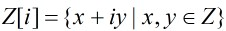叫做高斯整数环，其中Z表示通常的整数环，而用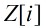表示复数域上的整数环。

那么什么是环呢？就是通过加减乘三种运算后，仍然能满足本身性质的就叫做环。

范的定义：设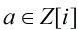，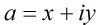，定义a的范为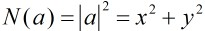设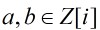，则

(1)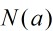为非负整数，并且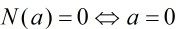(2)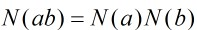(3)若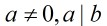，则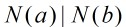逆的定义：设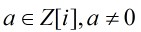，如果存在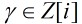，使得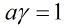，则称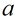为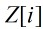中的乘法可逆元，简称可逆元，并且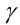叫做的逆。

高斯整数是可逆元的充要条件是：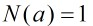。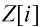中只有4个可逆元，分别是：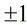和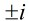定义：设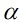和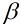是两个非零高斯整数，如果存在可逆元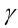，使得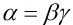，则称和等价，并表示成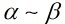,换句话说，与等价，是指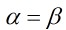，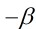，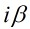或者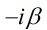高斯素数
定义：设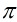为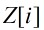中的非零非可逆元，我们称为高斯素数，是指的每个因子或者为可逆元，或者是与等价的高斯整数。

引理：
(1)设为高斯整数，并且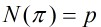为素数，则必定为高斯素数。
(2)若为高斯素数，则其共轭元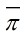也是高斯素数。

如何判断一个高斯整数是否属于高斯素数呢？可以用下面的方法：

高斯整数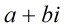是素数当且仅当：
(1)a、b中有一个是零，另一个数的绝对值是形如4n+3的素数；
(2)a、b均不为零，而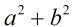为素数；

有了这个结论，那么我们就可以很轻松的解决HDU2650题了。

题目：A math problem

题意：给出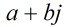，其中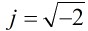，判断是否为高斯素数。

分析：其实就是上面的判断高斯素数的方法，但是注意一点，这里，而正常情况是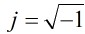，其实差不多一样，
只是把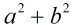为素数这个条件改为：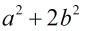为素数即可，那么如果把题目描述改为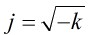呢？同样的道理只需把
判断条件改成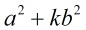为素数即可，由于很大，所以写个Miller_Rabin吧。。。

#include <stdio.h>
#include <stdlib.h>
#include <string.h>
#include <iostream>

const int Times=10;

using namespace std;
typedef long long LL;

LL multi(LL a,LL b,LL m)
{
LL ans=0;
while(b)
{
if(b&1)
{
ans=(ans+a)%m;
b--;
}
b>>=1;
a=(a+a)%m;
}
return ans;
}

LL quick_mod(LL a,LL b,LL m)
{
LL ans=1;
a%=m;
while(b)
{
if(b&1)
{
ans=multi(ans,a,m);
b--;
}
b>>=1;
a=multi(a,a,m);
}
return ans;
}

bool Miller_Rabin(LL n)
{
if(n==2) return true;
if(n<2||!(n&1)) return false;
LL a,m=n-1,x,y;
int k=0;
while((m&1)==0)
{
k++;
m>>=1;
}
for(int i=0;i<Times;i++)
{
a=rand()%(n-1)+1;
x=quick_mod(a,m,n);
for(int j=0;j<k;j++)
{
y=multi(x,x,n);
if(y==1&&x!=1&&x!=n-1) return false;
x=y;
}
if(y!=1) return false;
}
return true;
}

int main()
{
LL a,b;
while(~scanf("%I64d%I64d",&a,&b))
{
if(a==0)
{
if(b%4==3&&Miller_Rabin(b)) puts("Yes");
else  puts("No");
}
else
{
LL t=a*a+2*b*b;
if(Miller_Rabin(t)) puts("Yes");
else  puts("No");
}
}
return 0;
}


题目：http://poj.org/problem?id=3361

#include <iostream>
#include <stdlib.h>
#include <string.h>
#include <stdio.h>
#include <math.h>

using namespace std;
const int N=100005;

int prime[N], p[N],k;
int pri[N],top;
int n;

struct point
{
int a;
int b;
char oper;
}s[N];

int num;
//筛选素数

void isprime()
{
k=0;
int i,j;
memset(prime,true,sizeof(prime));
for(i=2;i<N;i++)
{
if(prime[i])
{
p[k++]=i;
for(j=i+i;j<N;j+=i)
{
prime[j]=false;
}
}
}
}

//素因子分解
void Divide(int n)
{
int i;
top=0;
for(i=0; i<k; i++)
{
if(n%p[i]==0)
{
pri[top++]=p[i];
n/=p[i];
while(n%p[i]==0)
{
pri[top++]=p[i];
n/=p[i];
}
}
}
if(n>1)
pri[top++]=n;
}

//高斯素数分解
void Part(int prime)
{
int i;
if(prime==2)
{
s[num].a = 1;
s[num].b = 1;
s[num++].oper = '+';
s[num].a = 1;
s[num].b = 1;
s[num++].oper = '-';
}
else if((prime - 1)%4==0)
{
for(i=1;;i++)
{
int u=int(sqrt(prime-i*i*1.0)+1e-5);
if(u*u+i*i==prime)
{
s[num].a = i;
s[num].b = u;
s[num++].oper='+';
s[num].a = i;
s[num].b = u;
s[num++].oper='-';
break;
}
}
}
else
{
s[num].a=prime;
s[num++].b=0;
}
}

int cmp(const void *a, const void *b)
{
point *c = (point *)a;
point *d = (point *)b;
if(c->a != d->a)
return c->a - d->a;
if(c->b != d->b)
return c->b - d->b;
return c->oper == '-' ? 1 : -1;
}

void Print(int key)
{
printf("%d", s[key].a );
if(s[key].b == 0)
return;
if(s[key].b == 1)
printf("%cj", s[key].oper);
else
printf("%c%dj", s[key].oper, s[key].b);
}

int main()
{
isprime();
int i, cas=1;
while(~scanf("%d", &n))
{
num = 0;
Divide(n);
for(i=0;i<top;i++)
Part(pri[i]);
qsort(s, num, sizeof(point), cmp);
printf("Case #%d: ", cas++);
Print(0);
for(i=1; i<num; i++)
{
if(s[i].a==s[i-1].a
&&s[i].b==s[i-1].b
&&s[i].oper==s[i-1].oper)
continue;
if(i)
printf(", ");
Print(i);
}
puts("");
}
return 0;
}

展开全文• First complex number: 471 + 643i不是高斯素数 Second complex number: 9 + 11i不是高斯素数 The sum of the two numbers: 480 + 654i The Minus of the two numbers: 462 + 632i The Product of the two numbers: ...
• 我们把集合：叫做高斯整数环，其中Z表示通常的整数环，而用表示复数域上的整数环。   那么什么是环呢？就是通过加减乘三种运算后，仍然能满足本身性质的就叫做环。     范的定义：设，，定义a的范...
• #include #include #include #include using namespace std; int ok(int x) { for(int i=2;i(int)sqrt(x+0.5);i++) { if(x%i==0) return 0; } return 1; } int main() { int T,a,b;... wh
• 在这里，我们展示了编码为 lcf 的 mimo 系统的 ber 仅取决于高斯整数的最小多项式。 这是我们的分钟。 2x2 系统的方程是 x^2+i=0（其中 i 是复数 i） 两个不同的根集是 a.exp((-1i*pi)/4),exp((-1i*5*pi)/4) b.exp...matlab
• http://zh.wikipedia.org/wiki/高斯整數 转载于:https://www.cnblogs.com/luotinghao/archive/2012/11/22/2782434.html
• 我们把集合：叫做高斯整数环，其中Z表示通常的整数环，而用表示复数域上的整数环。   那么什么是环呢？就是通过加减乘三种运算后，仍然能满足本身性质的就叫做环。     范的定义：设，，定义a的范...
• 数学家高斯的问题，一个有意思的小算法，根据整数计算日期
• 对互为共轭复数的高斯整数，将其分成两部分，每一对共轭复数留一个在左边，另一个留在右边，最后左边的高斯整数相乘得到的高斯整数和右边高斯整数相乘得到的高斯整数互为共轭复数，并且它们的乘积 = z = z = z ...高斯质数 圆上整点
• <br /> <br />注：这是从新浪网转载过来的 链接:http://blog.sina.com.cn/s/blog_6e3d28f70100n64y.html
• 高斯消元就是来接方程组的。（可以跟矩阵联系在一起）#include #include #include #include #include using namespace std; const int MAXN = 1e2+5; int equ, var;///equ个方程 var个变量 int a[MA
• #include #include #include using namespace std; const int MAXN = 100; int mabs(int u){return u>0?u:-u;} int gcd(int a,int b){ int c; while(b){ c = a;c++ 算法
• #include #include #include using namespace std; #define MAXN 400 int a[MAXN+10][MAXN+10],x[MAXN+10],n,m,row; template void Read(T &x){ char c; bool f=0;c++ noi 算法
• 【信号、图像、Matlab】如何得到高斯滤波器的整数模板如何得到高斯滤波器的整数模板？这个问题困扰了我两天，上网搜索的代码，基本上都生成的小数，有的文档给写了3*3，5*5，7*7的整数形式，但是没有说是怎么得到的...信号 图像 Matlab...